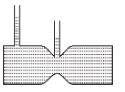Courses

# Fluid Dynamics MCQ Level - 2

## 10 Questions MCQ Test Topic wise Tests for IIT JAM Physics | Fluid Dynamics MCQ Level - 2

Description
This mock test of Fluid Dynamics MCQ Level - 2 for IIT JAM helps you for every IIT JAM entrance exam. This contains 10 Multiple Choice Questions for IIT JAM Fluid Dynamics MCQ Level - 2 (mcq) to study with solutions a complete question bank. The solved questions answers in this Fluid Dynamics MCQ Level - 2 quiz give you a good mix of easy questions and tough questions. IIT JAM students definitely take this Fluid Dynamics MCQ Level - 2 exercise for a better result in the exam. You can find other Fluid Dynamics MCQ Level - 2 extra questions, long questions & short questions for IIT JAM on EduRev as well by searching above.
QUESTION: 1

### Water flows steadily from an tank as shown. Find x where the water lands. Assume area of cross-section of hole is much less than area of cross-section of tank.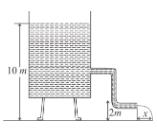Solution: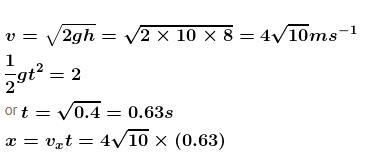= 2.53 (3.162) = 7.97
The correct answer is: 7.97 m

QUESTION: 2

### A cylindrical container of an incompressible liquid rotates with ω as shown. The density of liquid is ρ. The liquid acquires parabolic shape. Find the height of the liquid. Radius of container is r.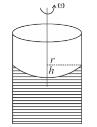Solution:

The force experienced by element dx at distance x from the centre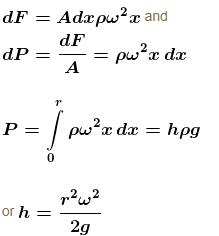Note : This technique is employed to make parabolic telescopic mirrors.

Liquid glass is rotated and allowed to solidify while rotating.

The correct answer is: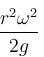QUESTION: 3

### A shower head has 20 circular opening each of radius 1 mm. Shower head is connected to a pipe of radius 0.8 cm. The speed of water in the pipe is 3ms–1. Find the speed of water as it exists from the shower openings.

Solution: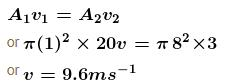The correct answer is: 9.6 ms–1

QUESTION: 4

A cylindrical container of radius R and height h is completely filled with a liquid. Two horizontal L shaped pipes of small cross-section area a are connected to the cylinder as shown in the figure. Now the two pipes are opened and fluid starts coming out of the pipes horizontally in opposite directions. Then the torque due to ejected liquid on the system is :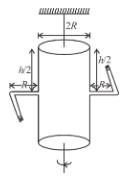Solution:

Velocity of efflux of water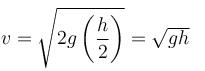force on ejected water = Rate of change of momentum of ejected water.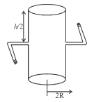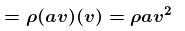Torque of these forces about central line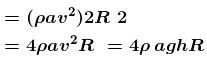The correct answer is: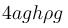QUESTION: 5

A steady stream of water falls straight down from a pipe as shown. Assume the flow is incompressible, then :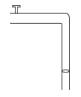Solution: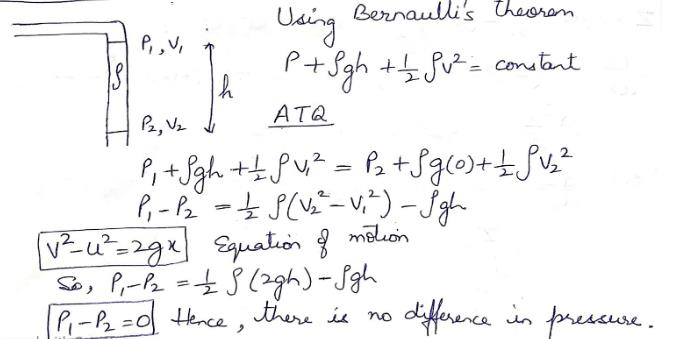QUESTION: 6

A narrow tube completely filled with a liquids lying on a series of cylinders as shown in figure. Assuming no sliding between any surfaces, the value of acceleration of the cylinders for which liquids will not come out of the tube from anywhere is given by :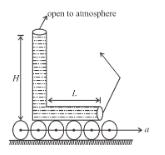Solution:

No sliding ⇒ pure rolling

Therefore, acceleration of the tube = 2a (since centre of mass of cylinders are moving at a)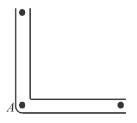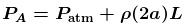(From horizontal limb)

Also;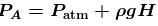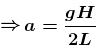The correct answer is: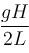QUESTION: 7

A non uniform cylinder of mass m, length l and radius r is having its centre of mass at a distance l/4 from the centre and lying on the axis of the cylinder. The cylinder is kept in a liquid of uniform density ρ. The moment of inertia of the rod about the centre of mass is I. The angular acceleration of point A relative to point B just after the rod is released from the position shown in figure is :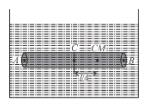Solution: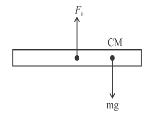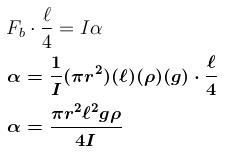α will be same for all points.

The correct answer is: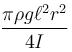QUESTION: 8

U-tube of base length ℓ filled with same volume of two liquids of densities ρ and 2ρ is moving with an acceleration a on the horizontal plane. If the height difference between the two surfaces (open to atmosphere) becomes zero, then the height  h  is given by :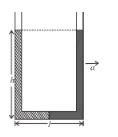Solution:

For the given situation, liquid of density 2ρ should be behind that of ρ.

From right limb :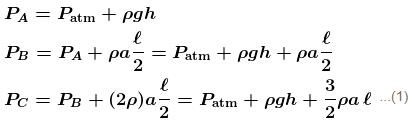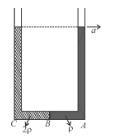But from left limb :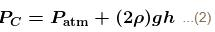From (1) and (2) :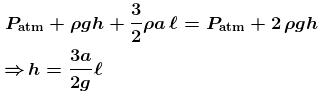The correct answer is: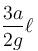QUESTION: 9

A cylindrical vessel filled with water is released on an inclined surface of angle θ as shown in figure. The friction coefficient of surface with vessel is μ (< tan θ.) Then the constant angle made by the surface of water with the incline will be :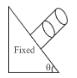Solution:

Since frictional force = pulling force

i.e. μmgcosθ = mgsinθ

tanθ = μ

θ = tan-1μ​

here θ is angle made by surface of water with incline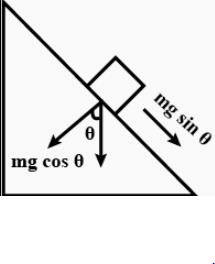QUESTION: 10

For a liquid which is following steadily, the level in the vertical tubes is best represented by :

Solution:

From continuity equation, velocity at cross-section (1) is more than that at cross-section (2).

Hence ; P1 < P2
The correct answer is: